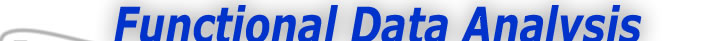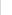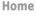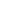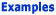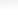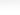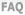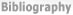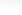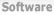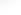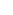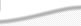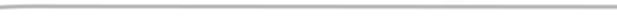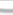BasisFunctions Goods -The Energy in the Nondurable Goods Index -The Phase-plane Plot of Acceleration Versus Velocity -Plotting the Depression and World War II -The Mid 70's: A time of Structural Change -What have we seen? Growth Weather
 Phase-Plane Plotting of the Goods Index Expertise: Intermediate The Phase-plane Plot of Acceleration Versus Velocity This little physics lesson suggests that we should plot acceleration against velocity to study the trade-off between these two types of energy. Figure 2 shows us what we see if we do this for the pendulum; the plot is an ellipse, with potential energy being reflected in the vertical axis and kinetic energy associated with the horizontal axis.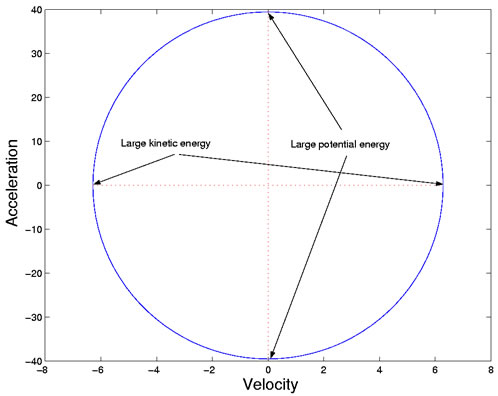Figure 2: The plot of acceleration against velocity, or a phase-plane plot, for a swinging pendulum. We use the phase-plane plot, therefore, to study the energy transfer within the economic system. We can examine the cycle within individual years, and also see more clearly how the structure of the transfer has changed throughout the twentieth century. Figure 3 phase-plane plots the year 1964, a year in a relatively stable period for the index. To read the plot, find the lower-case "j" in the middle right of the plot, and move around the diagram clockwise, noting the letters indicating the months as you go. You will see that there are two large cycles surrounding zero, plus some small cycles that are much closer to the origin.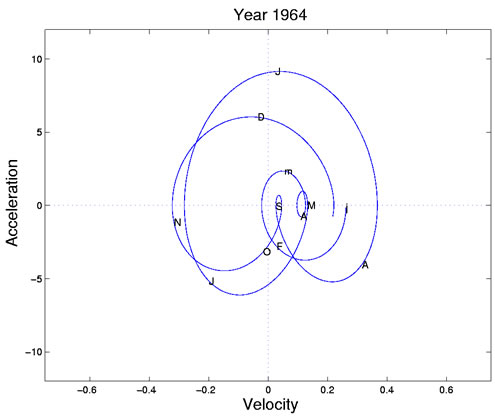Figure 3: A phase-plane plot of the first derivative or velocity and the second derivative or acceleration of the smoothed log nondurable goods index for 1964. Letters indicate mid-months, with lowercase letters used for January and March. The largest cycle begins in mid-May (M), with positive velocity but near zero acceleration. Production is increasing linearly or steadily at this point. The cycle moves clockwise through June (first J) and passes the horizontal zero acceleration line at the end of the month, when production is now decreasing linearly. By mid-July (second J) kinetic energy or velocity is near zero because vacation season is in full swing. But potential energy or acceleration is high, and production returns to the positive kinetic/zero potential phase in early August (A), and finally concludes with a cusp at summer's end (S). At this point the process looks like it has run out of both potential and kinetic energy. The cusp, near where both derivatives are zero, corresponds to the start of school in September, and to the beginning of the next big production cycle passing through the autumn months of October through November. Again this large cycle terminates in a small cycle with little potential and kinetic energy. This takes up the months of February and March (F and m). The tiny sub-cycle during April and May seems to be due to the spring holidays, since the summer and fall cycles, as well as the cusp, don't change much over the next two years, but the spring cycle cusp moves around, reflecting the variability in the timings of Easter and Passover. To summarize, the production year in the 1960s has two large cycles swinging widely around zero, each terminating in a small cusp-like cycle. This suggests that each large cycle is like a balloon that runs out of air, the first at the beginning of school, and the second at the end of winter. At the end of each cycle, it may be that new resources must be marshalled before the next production cycle can begin.